Expressions## Expressions

بازدید : 615 سه شنبه 14 بهمن 1393

# Expressions

A grads expression consists of operators, operands, and parentheses. Parentheses are used to control the order of operation.

Operators are:

`+`    Addition
``` -    Subtraction  `````` *    Multiplication  `````` /    Division ```

Operands are:

`variable specifications, functions, and constants`.

Operations between two variables are done on equivalent grid points in each grid. Missing data values in either grid give a result of a missing data value at that grid point. Dividing by zero gives a result of a missing data value at that grid point.

Operations cannot be done between grids that have different scaling in their varying dimensions -- i.e., grids that have different rules for converting the varying dimensions from grid space to world coordinate space. This can only be encountered when you are attempting operations between grids from different files that have different scaling rules.

If one grid has more varying dimensions than the other, the grid with fewer varying dimensions is 'expanded' and the operation is performed.

Expression evaluation in grads is recursive, so that multiple expressions may be nested together.

(grads version 2.0.a7+) Variable specifications can include a dimension expression to set time as an offset from the variable's initial time.

Some examples of expressions:

`slp/100                     `(Convert sea level pressure units from hPa to mb)
``` z-z(t-1)                    (Height change over one time step) `````` z-z(offt=0)                 (Height change since initial time) `````` t(lev=500)-t(lev=850)       (Temp difference between 500 and 850mb) `````` ave(z,t=1,t=5)              (Average of z over first 5 times in file)  `````` sum(prec(offt+0),t=1,t=4)   (Accumulated precipitation -- sum of 2nd through 5th time steps in file)  `````` z-ave(z,lon=0,lon=360,-b)   (Remove zonal mean) `````` tloop(aave(p,global))       (Time series of globally averaged precip) ```
مطالب مرتبط
ارسال نظر برای این مطلب

نام
ایمیل (منتشر نمی‌شود) (لازم)
وبسایت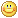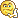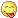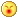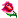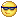نظر خصوصی
مشخصات شما ذخیره شود ؟ [حذف مشخصات] [شکلک ها]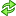کد امنیتی
اطلاعات کاربری
 نام کاربری : رمز عبور :
• فراموشی رمز عبور؟
• لینک دوستان
آرشیو
آمار سایت
• کل مطالب : 155
• کل نظرات : 0
• افراد آنلاین : 1
• تعداد اعضا : 4
• آی پی امروز : 8
• آی پی دیروز : 14
• بازدید امروز : 46
• باردید دیروز : 66
• گوگل امروز : 0
• گوگل دیروز : 0
• بازدید هفته : 295
• بازدید ماه : 2,481
• بازدید سال : 34,319
• بازدید کلی : 155,371
• کدهای اختصاصی